# Theory and Applications

### SSP Shen and RCJ Somerville

Version 1.0 released in July 2019 and coded by Dr. Samuel Shen, Distinguished Professor
San Diego State University, California, USA
Email:

Version 2.0 compiled and updated by Momtaza Sayd
San Diego State University May 2021
Version 3.0 compiled and updated by Joaquin Stawsky
San Diego State University June 2022

## Chapter 3: Basic Statistical Methods for Climate Data Analysis

### Mean, variance, standard deviation, skewness, kurtosis, and quantiles

``````#R can directly read data from a remote data website
setwd("~/sshen/climmath/data") #set the working directory
dim(dat1)``````
``##  136   6``
``````tmean15 <- dat1[,2] #Take only the second column of this data matrix
``##  -0.367918 -0.317154 -0.317069 -0.393357 -0.457649 -0.468707``
``````# -0.367918 -0.317154 -0.317069 -0.393357 -0.457649 -0.468707
mean(tmean15)``````
``##  -0.2034367``
``````# -0.2034367
sd(tmean15)``````
``##  0.3038567``
``````# 0.3038567
var(tmean15)``````
``##  0.09232888``
``````# 0.09232888
library(e1071) ``````
``## Warning: package 'e1071' was built under R version 4.1.3``
``````#This R library is needed to compute the following parameters
#install.packages("e1071") #if it is not installed on your computer
skewness(tmean15)``````
``##  0.7141481``
``````# 0.7141481
kurtosis(tmean15)``````
``##  -0.3712142``
``````# -0.3712142
median(tmean15)``````
``##  -0.29694``
``````# -0.29694
quantile(tmean15,probs = c(0.05,0.25, 0.75, 0.95))``````
``````##         5%        25%        75%        95%
## -0.5792472 -0.4228540 -0.0159035  0.3743795``````
``````#        5%        25%        75%        95%
#-0.5792472 -0.4228540 -0.0159035  0.3743795 ``````

### Correlation, covariance, and linear trend

``````#Plot Fig. 3.1
yrtime15 <- seq(1880, 2015)
reg8015 <- lm(tmean15 ~ yrtime15)
# Display regression results
reg8015``````
``````##
## Call:
## lm(formula = tmean15 ~ yrtime15)
##
## Coefficients:
## (Intercept)     yrtime15
##  -13.208662     0.006678``````
``````par(mar = c(4,4,4,1))
# Plot the temperature time series and its trend line
plot(yrtime15,tmean15,xlab = "Year",ylab = "Temperature [°C]",
main = "Global Annual Mean Land and Ocean Surface\nTemperature Anomalies 1880-2015", type = "l", lwd = 2,
cex.lab = 1.1,cex.axis = 1.1,cex.main = 1.35)
abline(reg8015, col = "red")
text(1932, 0.4, "Linear Temperature Trend 0.67 °C/century",
col = "red",cex = 1.1)``````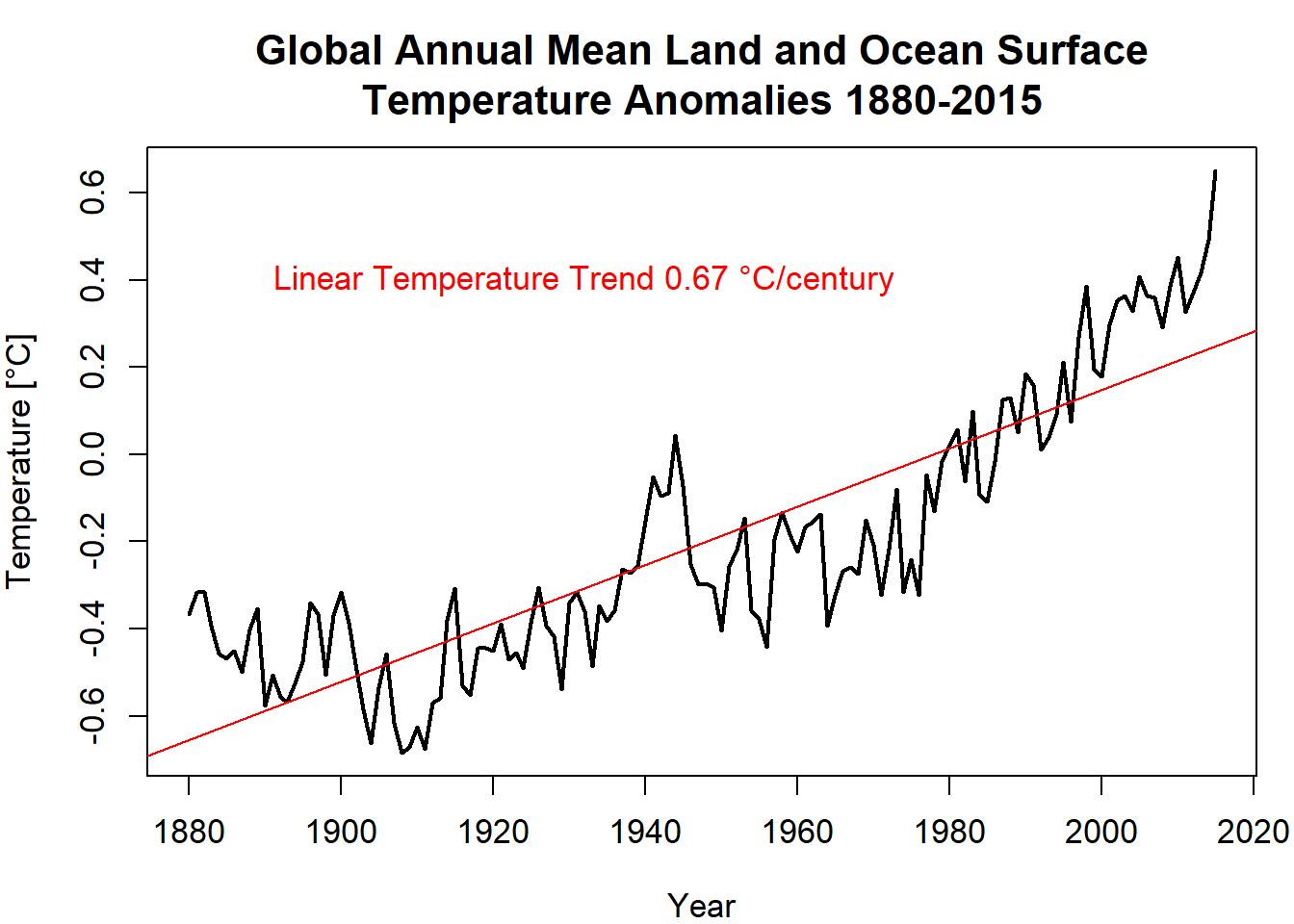``````#Covariance
x <- yrtime15
y <- tmean15
cov(x, y)``````
``##  10.36856``
``````#Correlation
cor(tmean15, yrtime15)``````
``##  0.8659857``
``````#Verify this result by formulas
x <- yrtime15
y <- tmean15
sx <- sd(yrtime15)
sy <- sd(tmean15)
cxy <- cov(x,y)
rxy <- cxy/(sx*sy)
#The same result as cor(tmean15, yrtime15)
rxy``````
``##  0.8659857``
``````#Verify the linear trend by formulas
rxy*sy/sx #verified``````
``##  0.006677908``
``cxy/sx^2 #verified``
``##  0.006677908``

### Histogram of a set of data

``````h <- hist(tmean15, main = "Histogram of 1880-2015 Temperature Anomalies",
xlab = expression(paste("Temperature Anomalies [", degree, "C]")),
xlim = c(-1,1), ylim = c(0,50))
xfit <- seq(-1,1, length = 100)
areat <- sum((h\$counts)*diff(h\$breaks[1:2]))#Normalization area
#diff(h\$breaks[1:2]) <- h\$breaks - h\$breaks
#is the histogram's bin width
yfit <- areat*dnorm(xfit, mean = mean(tmean15), sd = sd(tmean15))
#Plot the normal fit on the histogram
lines(xfit,yfit,col = "blue",lwd = 2,cex.lab = 1.1,cex.axis = 1.1,cex.main = 1.35)``````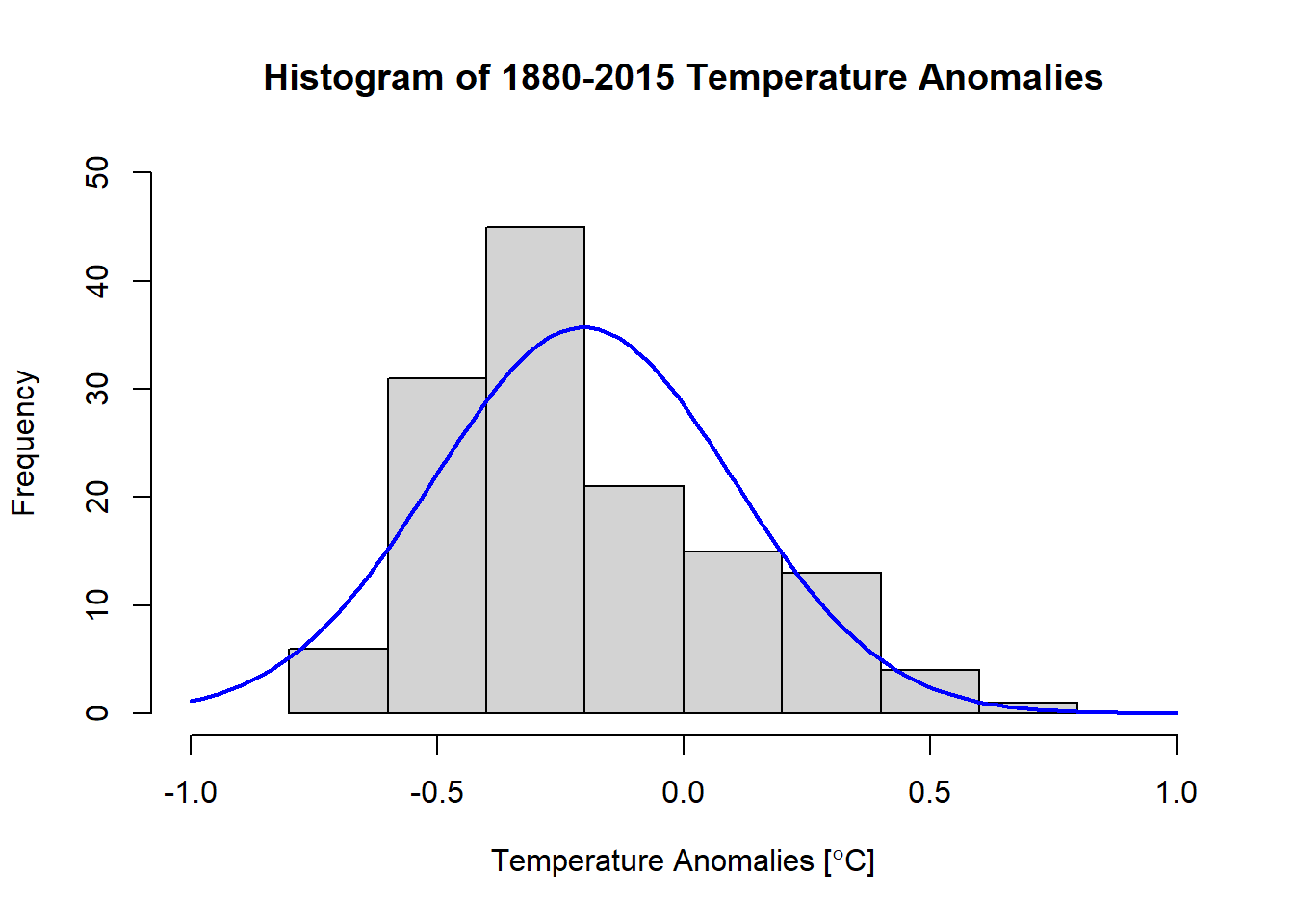### Box plot

``````boxplot(tmean15, ylim = c(-0.8,0.8),
ylab = expression(paste("Temperature Anomalies [", degree, "C]")),
cex.lab = 1.1)``````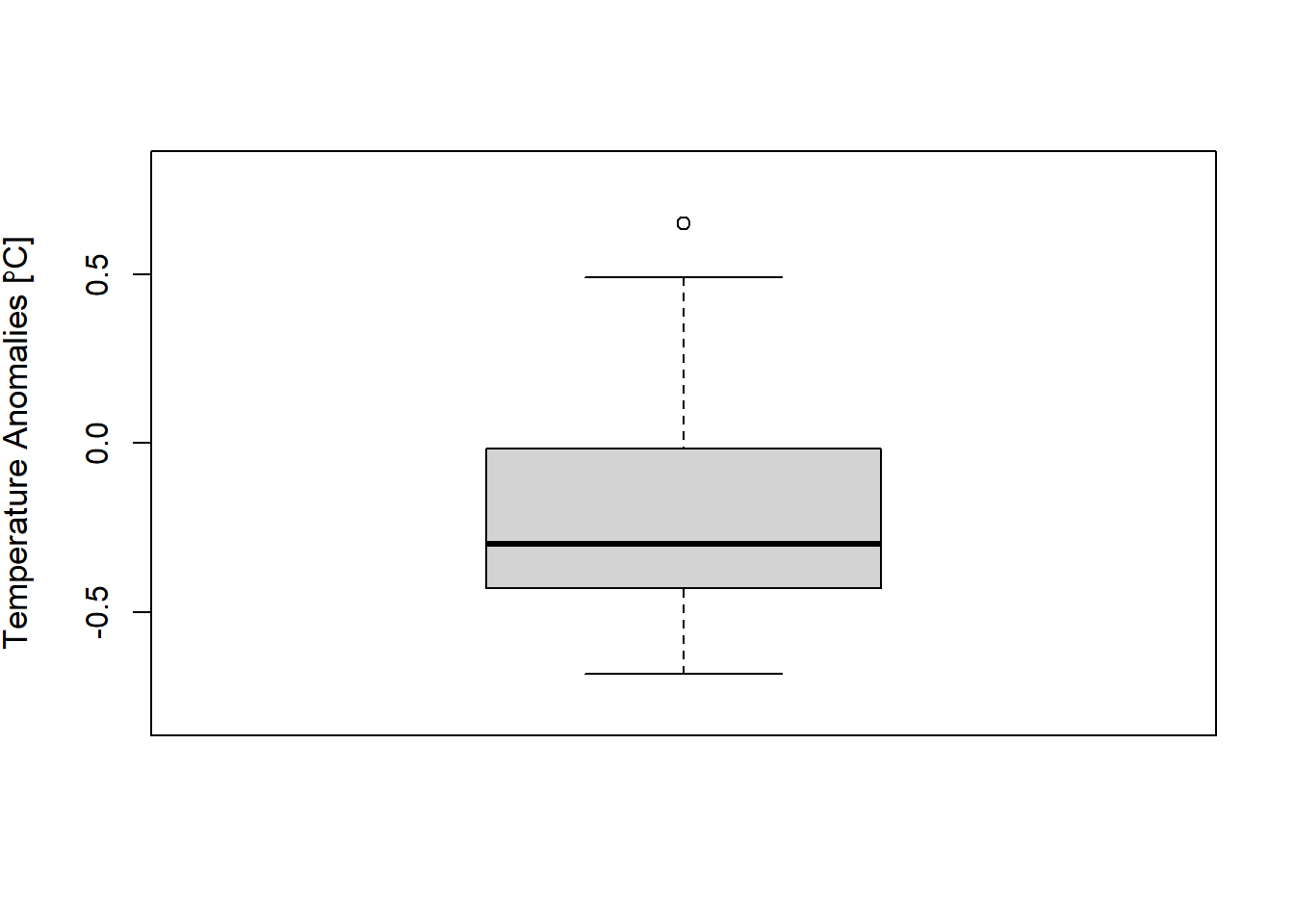### Plot Fig. 3.4

``````#Use setwd("working directory") to work in the desired directory
#rm(list = ls())
setwd("~/sshen/climmath/data")
par(mgp = c(1.5,0.5,0))
soid <- soi[,2] #Take the second column SOI data
soim <- matrix(soid,ncol = 12,byrow = TRUE)
#Make the SOI into a matrix with each month as a column
soij <- soim[,1] #Take the first column for Jan SOI
ustj <- ust[,3] #Take the third column: Jan US temp data
setEPS()
# postscript("fig0304.eps", height = 7, width = 7)
par(mar = c(4.5,5,2.5,1), xaxs = "i", yaxs = "i")
plot(soij,ustj,xlim = c(-4,4), ylim = c(-8,8),
main = "January SOI and the U.S. Temperature Anomalies",
xlab = "SOI [Dimensionless]",
ylab = expression(paste("Temperature Anomalies [", degree, "F]")),
pch = 19, cex.lab = 1.1,cex.axis = 1.1,cex.main = 1.35)
#Plot the scatter plot
soiust <- lm(ustj ~ soij) #Linear regression
abline(soiust, col = "blue", lwd = 4) #Linear regression line``````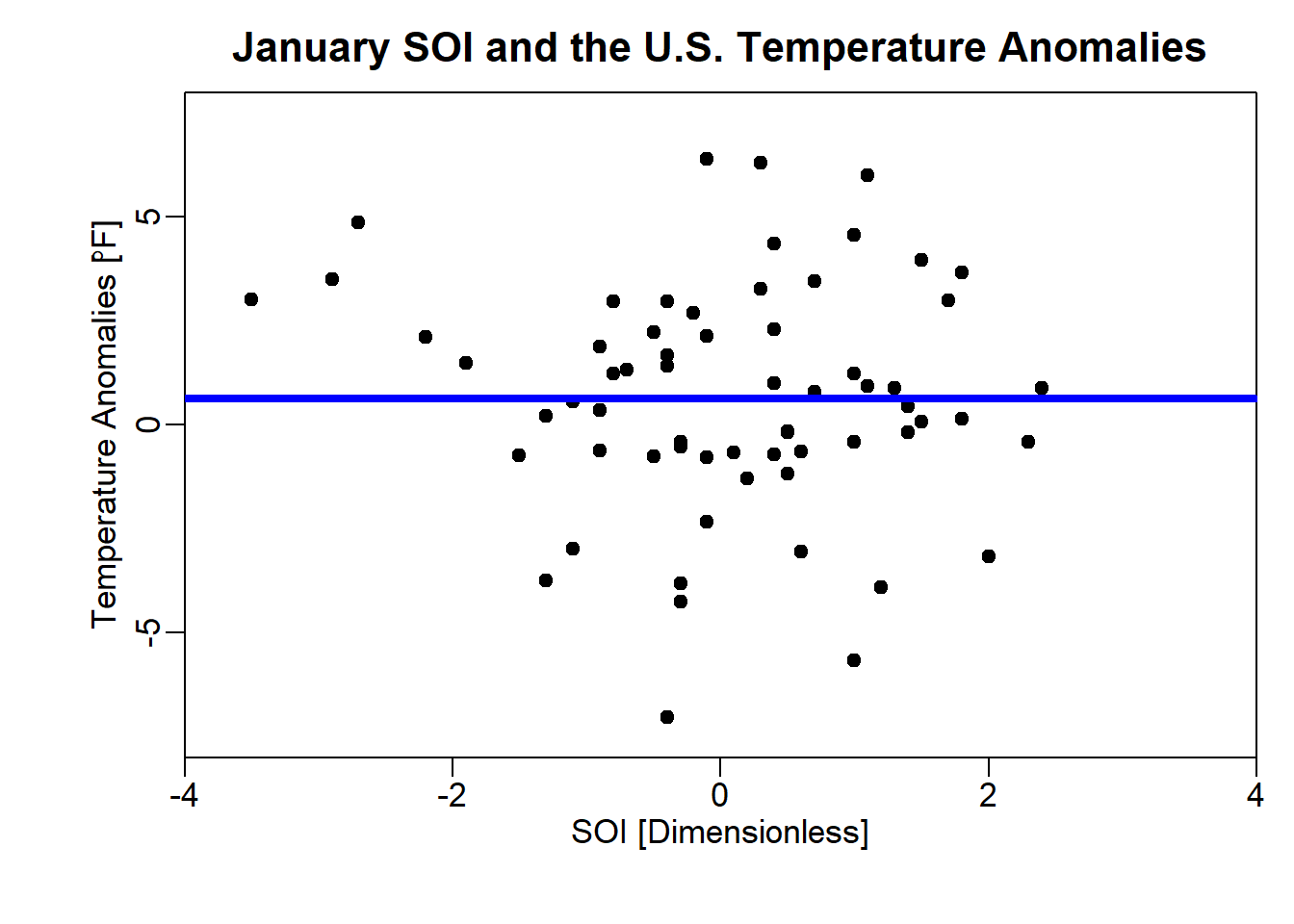``# dev.off()``

### Plot Fig. 3.5

``````#Q-Q plot for the standardized temperature anomalies
tstand <- (tmean15-mean(tmean15))/sd(tmean15)
set.seed(101)
qn <- rnorm(136) #Simulate 136 points by N(0,1)
qns <- sort(qn)#Sort the points
qq2 <- qqnorm(qns,col = "blue",lwd = 2,cex.lab = 1.1,cex.axis = 1.1,cex.main = 1.35)``````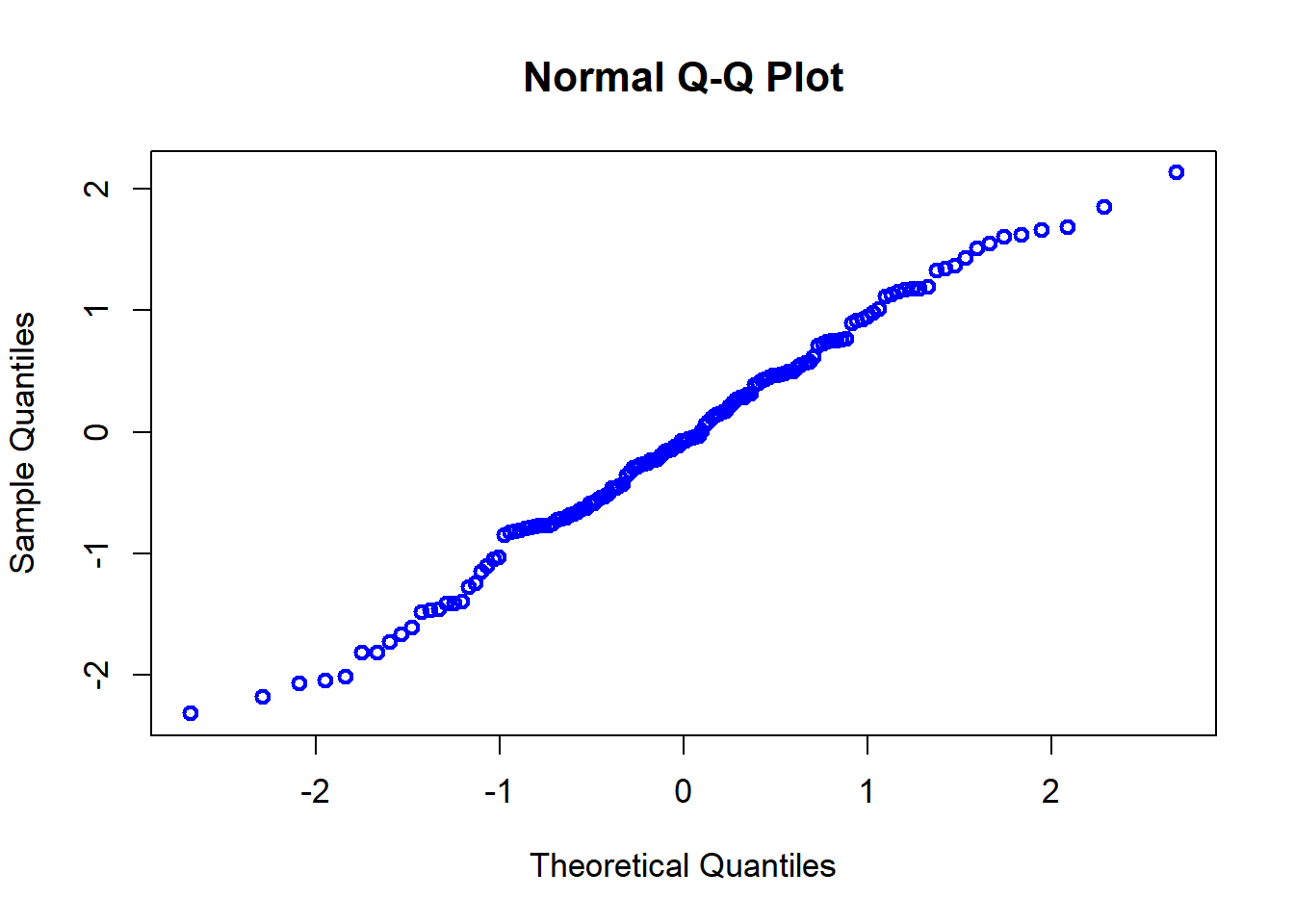``````setEPS()
# postscript("fig0305.eps", height = 7, width = 7)
par(mar = c(4.5,5,3,1), xaxs = "i", yaxs = "i")
qt <- qqnorm(tstand,
main = "Q-Q plot for the Standardized Global Temp.\n Anomalies vs N(0,1)",
ylab = "Quantile of Temperature Anomalies",
xlab = "Quantile of N(0,1)", xlim = c(-3,3),ylim = c(-3,3),
cex.lab = 1.1,cex.axis = 1.1,cex.main = 1.35)
qqline(tstand, col = "red", lwd = 3)
points(qq2\$x, qq2\$y, pch = 19, col = "purple")``````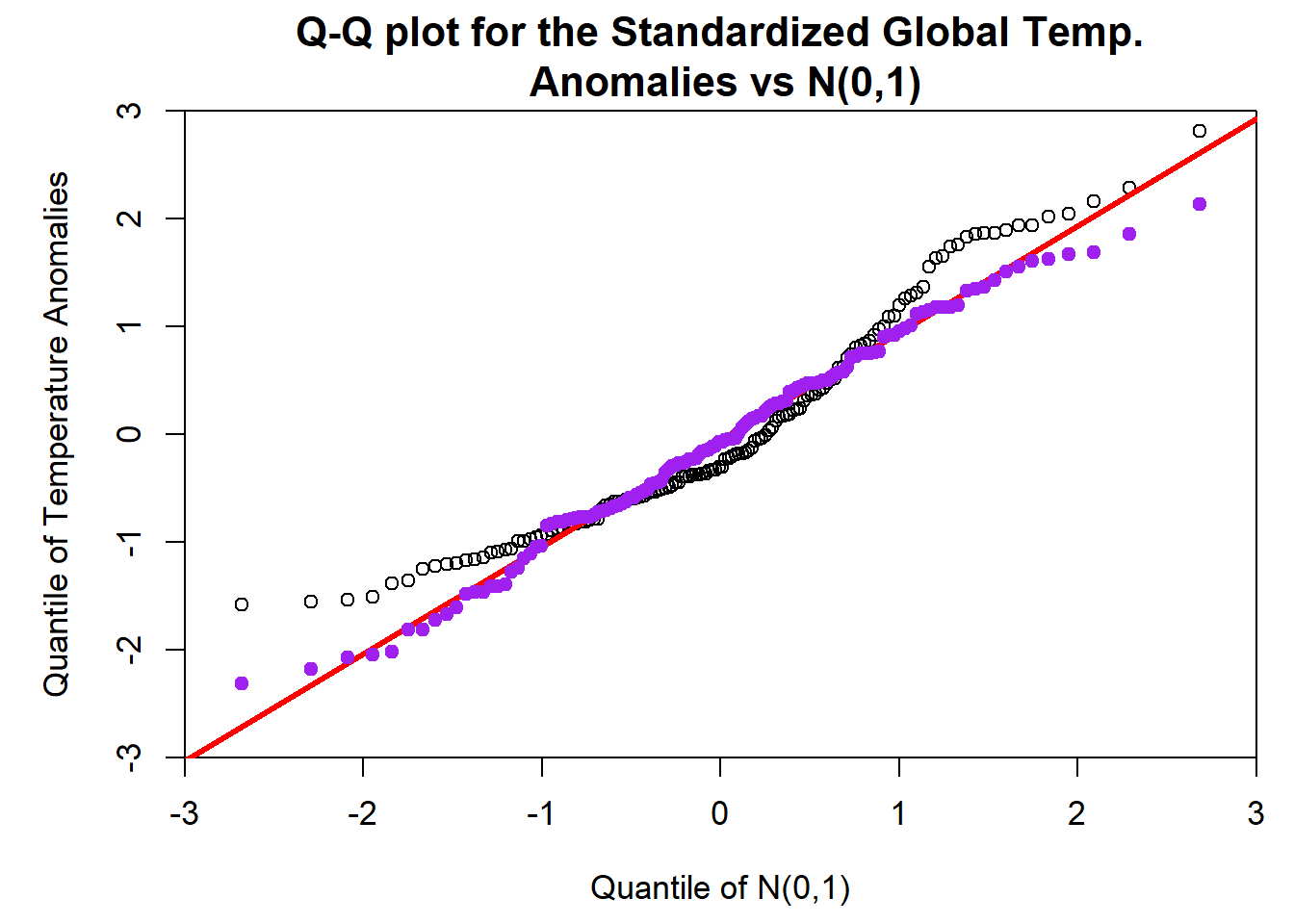``# dev.off()``

### Plot Fig. 3.6

``````plot.new()
layout(matrix(c(1,2,3), 1, 3, byrow = TRUE),
widths = c(3,3,3), heights = c(1,1,1))
lasvegas <- c(0.58,0.42)
sandiego <- c(0.4,0.6)
seattle <- c(0.16,0.84)
names(lasvegas) <- c("Clear","Cloudy")
names(sandiego) <- c("Clear","Cloudy")
names(seattle) <- c("Clear","Cloudy")
barplot(lasvegas,col = c("skyblue","gray"), ylim = c(0,1),
ylab = "Probability", cex.lab = 1.2)
mtext("Las Vegas", side = 3, line = 1)
barplot(sandiego,col = c("skyblue","gray"), ylim = c(0,1))
mtext("San Diego", side = 3, line = 1)
barplot(seattle,col = c("skyblue","gray"), ylim = c(0,1))
mtext("Seattle", side = 3, line = 1)
mtext(expression(bold("Probability Distribution of Cloudiness")),
cex = 1.2,side = 3, line = -1.5, outer = TRUE)``````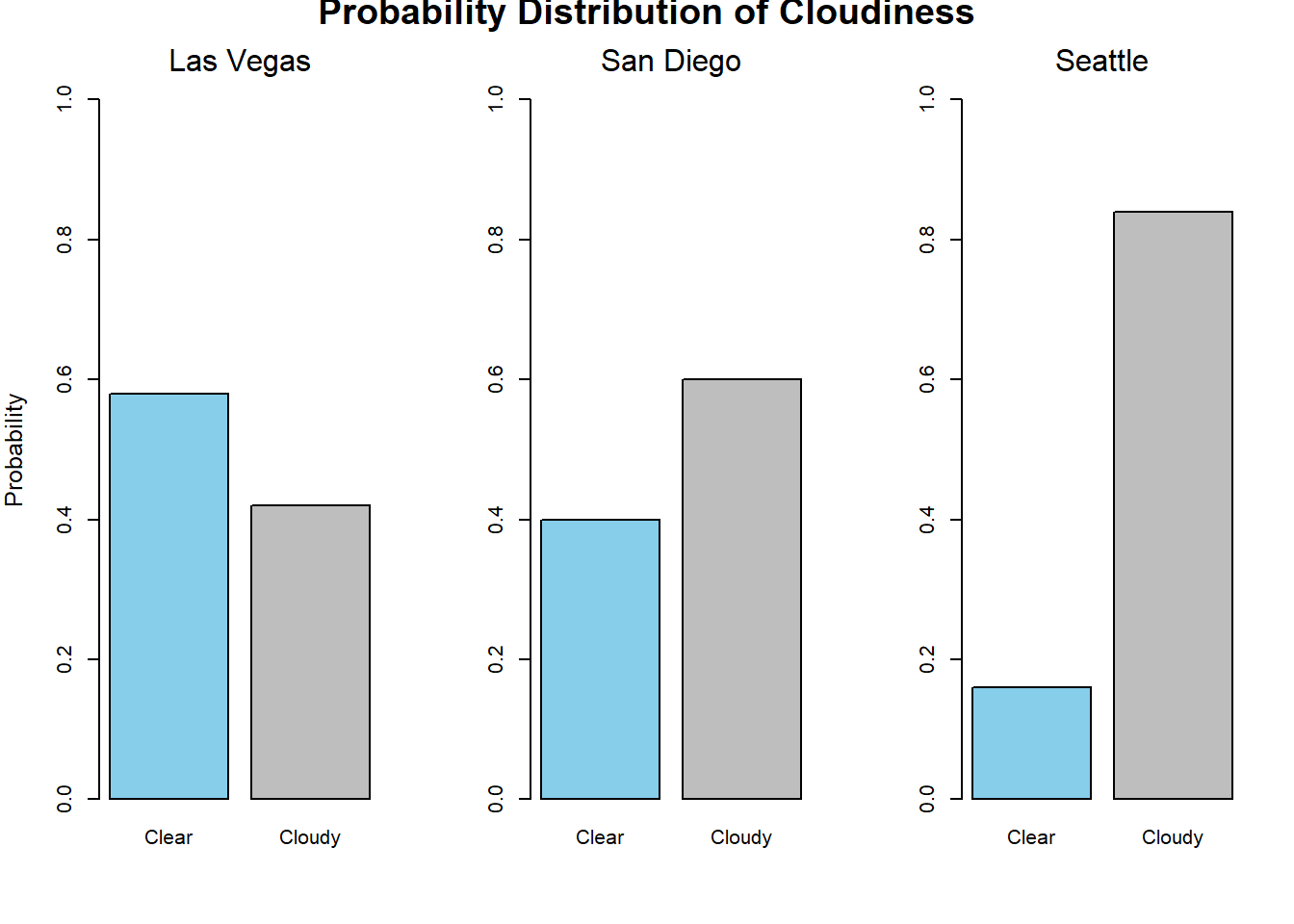### Plot Fig. 3.7

``````# Density function, probability, and normalization of N(0,1)
#par(mar = c(4.0,4.0,1.5,0.5))
cord.x <- c(-3,seq(-3,3,0.01),-1)
cord.y <- c(0,dnorm(seq(-3,3,0.01)),0)
# Make a curve
curve(dnorm(x,0,1), xlim = c(-3,3), lwd = 3,
main = 'PDF of the Standard Normal Distribution',
xlab = "Random Variable x",
ylab = 'Probability Density',
cex.lab = 1.1,cex.axis = 1.1,cex.main = 1.35)
polygon(cord.x,cord.y,col = 'skyblue')
polygon(c(-1.5,-1.5, -1.2, -1.2),c(0, dnorm(-1.5),
dnorm(-1.2), 0.0),col = 'white')
text(0,0.18, "Area = 1", cex = 1.1)
text(-1.65,0.045,"f(x)")
text(-1.35,0.075,"dx")
text(-1.6,0.0125,"x")
text(-0.9,0.0125,"x + dx")
arrows(-2,0.2,-1.35,0.13, length = 0.1)
text(-2,0.21,"dA = f(x)dx")
text(0,0.09,expression(paste(integral(f(x)*dx,- infinity,infinity)," = 1")))``````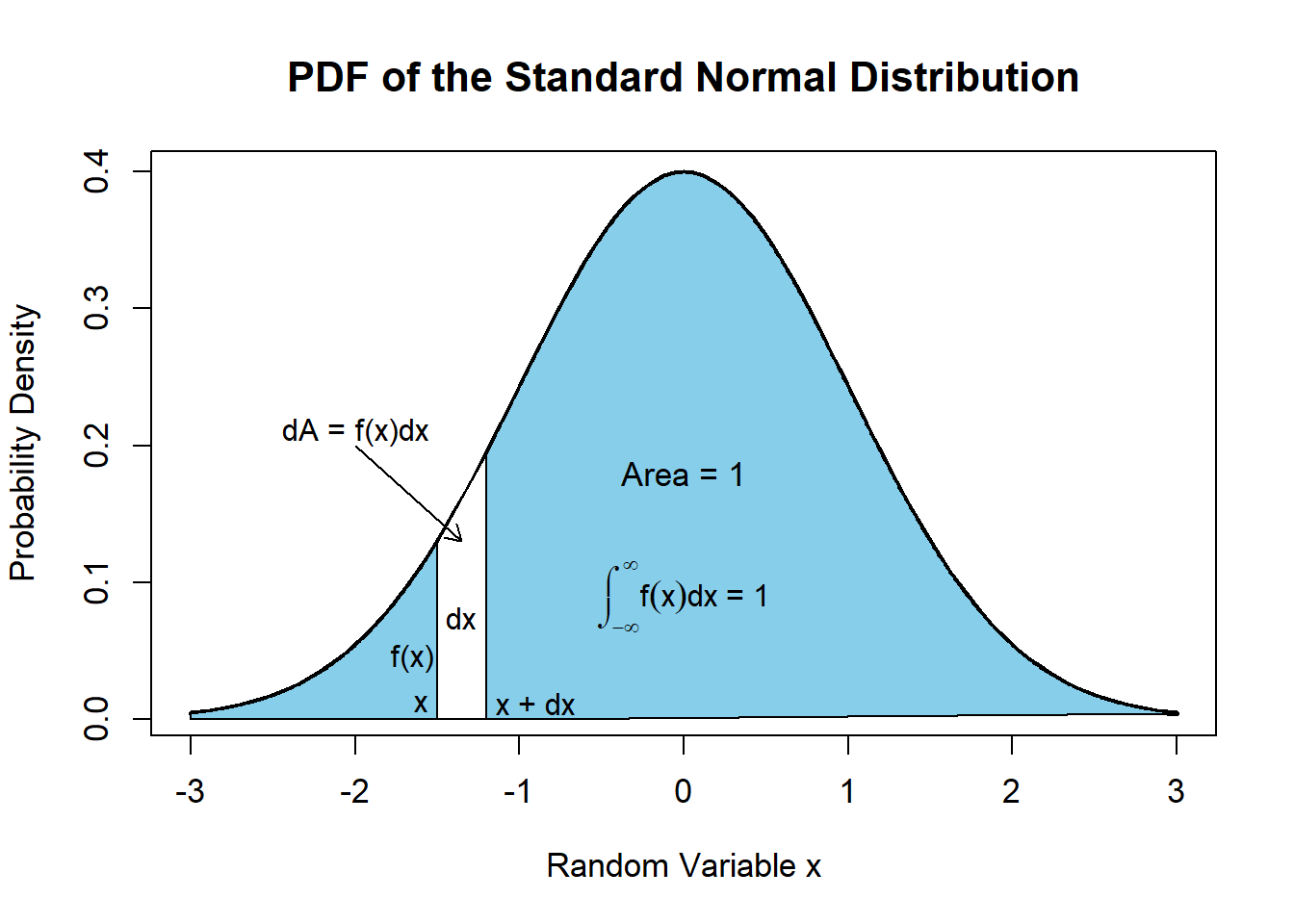### Plot Fig. 3.8

``````#Normal distribution plot
x <- seq(-8, 8, length = 200)
plot(x,dnorm(x, mean = 0, sd = 1), type = "l", lwd = 4, col = "red",
ylim = c(0,1),
xlab = "Random Variable x",
ylab = "Probability Density",
main = expression(Normal~Distribution ~ N(mu,sigma^2)))
lines(x,dnorm(x, mean = 0, sd = 2), type = "l", lwd = 2, col = "blue")
lines(x,dnorm(x, mean = 0, sd = 0.5), type = "l", lwd = 2, col = "black")
lines(x,dnorm(x, mean = 3, sd = 1), type = "l", lwd = 2, col = "purple")
lines(x,dnorm(x, mean = -4, sd = 1), type = "l", lwd = 2, col = "green")
# ex.cs1 <- expression(plain(sin) * phi,  paste("cos", phi))
ex.cs1 <- expression(paste(mu, " = 0",~","~ sigma," = 1"),
paste(mu, " = 0",~","~ sigma, " = 2"),
paste(mu, " = 0",~","~ sigma, " = 1/2"),
paste(mu, " = 3",~","~ sigma, " = 1"),
paste(mu, " = -4",~","~ sigma, " = 1"))
legend("topleft",legend = ex.cs1, lty = 1,
col = c('red','blue','black','purple','green'), cex = 1, bty = 'n')``````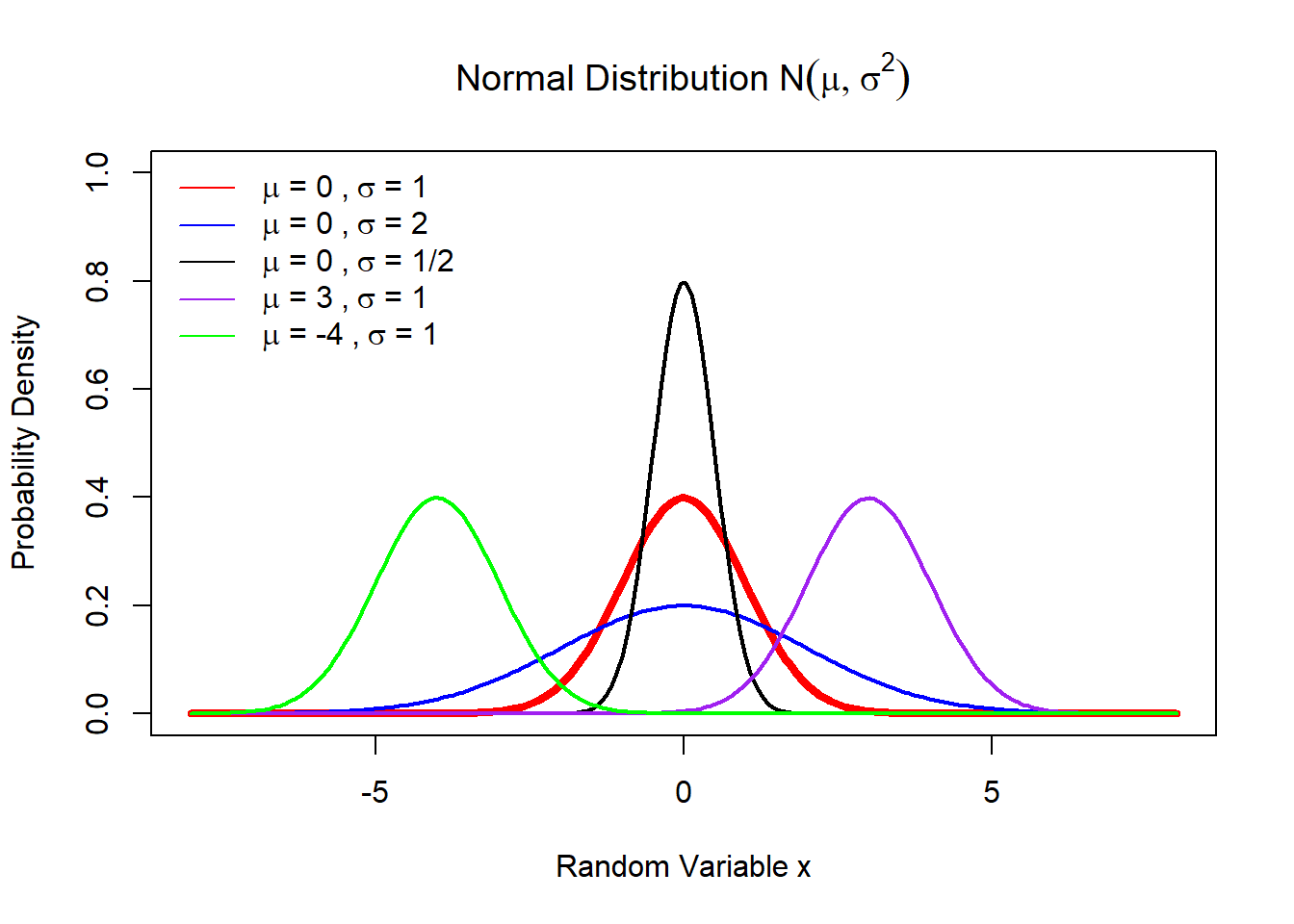### Calculate probability from a normal distribution

``````mu <- 0
sig <- 1
intg <- function(x){(1/(sig*sqrt(2*pi)))*exp(-(x-mu)^2/(2*sig^2))}
integrate(intg,-2,2)``````
``## 0.9544997 with absolute error < 1.8e-11``
``````#Or using the R built-in function dnorm to get the same result
integrate(dnorm,-2,2)``````
``## 0.9544997 with absolute error < 1.8e-11``
``integrate(dnorm,-1.96,1.96)``
``## 0.9500042 with absolute error < 1e-11``

### Plot Fig. 3.9

``````#Plot t-distribution by R
n <- 50
x <- seq(-4, 4, length = 200)
plot(x,dt(x, df = 3), type = "l", lwd = 4, col = "red",
ylim = c(0,0.6),
xlab = "Random Variable t",
ylab = "Probability Density",
main = "Student t-distribution T(t,df)",
cex.lab = 1.1,cex.axis = 1.1,cex.main = 1.35)
lines(x,dt(x, df = 1), type = "l", lwd = 2, col = "blue")
lines(x,dt(x, df = 2), type = "l", lwd = 2, col = "black")
lines(x,dt(x, df = 6), type = "l", lwd = 2, col = "purple")
lines(x,dt(x, df = Inf), type = "l", lwd = 2, col = "green")
#ex.cs1 <- expression(plain(sin) * phi,  paste("cos", phi))
ex.cs1 <- c("df = 3", "df = 1","df = 2","df = 6", expression(paste("df = ",infinity)))
legend("topleft",legend = ex.cs1, lty = 1,
col = c('red','blue','black','purple','green'), cex = 1, bty = "n")``````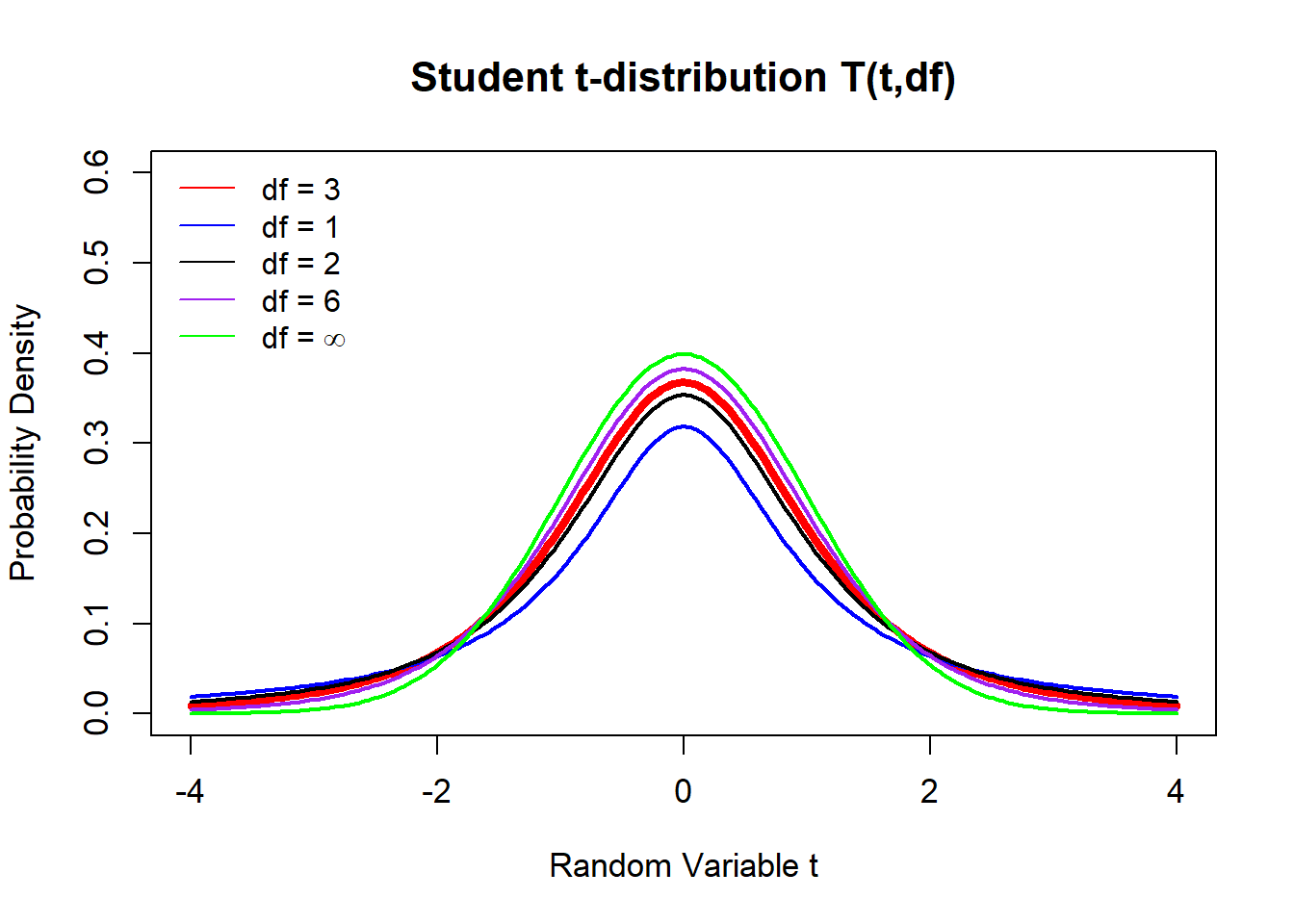### Confidence interval simulation

``````mu <- 14 #true mean
sig <- 0.3 #true sd
n <- 50 #sample size
d <- 1.96*sig/sqrt(n)
lowerlim <- mu-d
upperlim <- mu+d
ksim <- 10000 #number of simulations
k <- 0 #k is the simulation counter
xbar <- 1:ksim
for (i in 1:ksim)
{
xbar[i] <- mean(rnorm(n, mean = mu, sd = sig))
if (xbar[i] >= lowerlim & xbar[i] <= upperlim)
k <- k+1
}
print(c(k,ksim),cex.lab = 1.1,cex.axis = 1.1,cex.main = 1.35)``````
``##   9484 10000``

### Plot the histogram Fig. 3.10

``````hist(xbar,breaks = 51,
main = "Histogram of the Simulated\nSample Mean Temperatures",xaxt = "n",
xlab = expression(paste("Temperature [", degree, "C]")),
ylim = c(0,600),
cex.lab = 1.1,cex.axis = 1.1,cex.main = 1.35)
axis(1,pos = -20, at = c(13.92, mu, 14.08))
text(14,530,"95% Confidence Interval (13.92, 14.08)")``````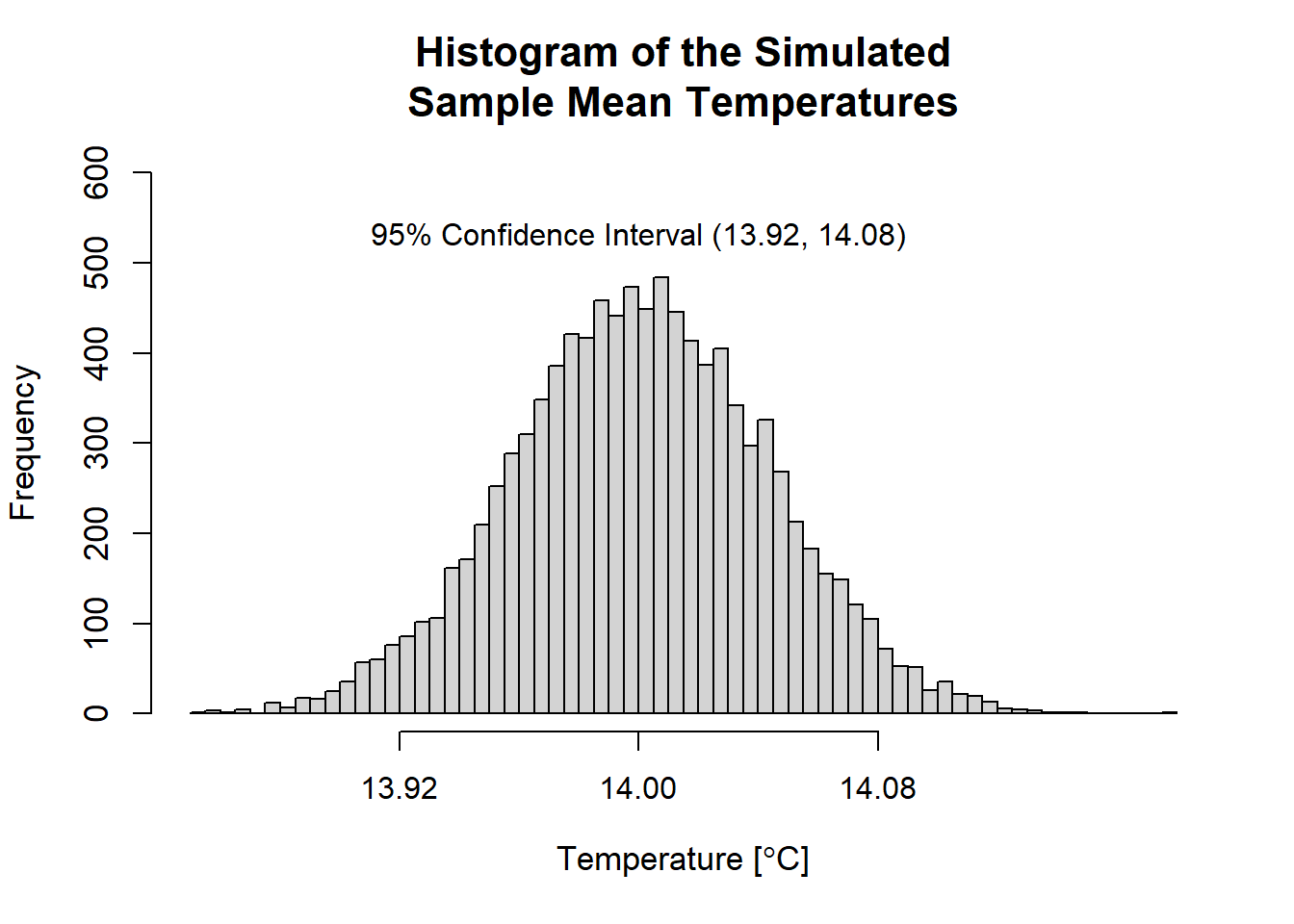### Plot Fig. 3.11

``````#Plot confidence intervals and tail probabilities
par(mar = c(2.5,3.5,2.0,0.5))
rm(list = ls())
par(mgp = c(1.4,0.5,0))
curve(dnorm(x,0,1), xlim = c(-3,3), lwd = 3,
main = 'Confidence Intervals and Confidence Levels',
xlab = "True Mean as a Random Variable", ylab = "",
xaxt = "n", cex.lab = 1.2)
title(ylab = 'Probability Density', line = 2, cex.lab = 1.2)
polygon(c(-1.96, seq(-1.96,1.96,len = 100), 1.96),
c(0,dnorm(seq(-1.96,1.96,len = 100)),0),col = 'skyblue')
polygon(c(-1.0,seq(-1.0, 1, length = 100), 1),
c(0, dnorm(seq(-1.0, 1, length = 100)), 0.0),col = 'white')
polygon(c(-3.0,seq(-3.0, -1.96, length = 100), -1.96),
c(0, dnorm(seq(-3.0, -1.96, length = 100)), 0.0),col = 'red')
polygon(c(1.96,seq(1.96, 3.0, length = 100), 3.0),
c(0, dnorm(seq(1.96, 3.0, length = 100)), 0.0),col = 'red')
points(c(-1,1), c(0,0), pch = 19, col = "blue")
points(0,0, pch = 19)
points(c(-1.96,1.96),c(0,0),pch = 19, col = "red")
text(0,0.02, expression(bar(x)), cex = 1.0)
text(-1.50,0.02, "SE", cex = 1.0)
text(-0.60,0.02, "SE", cex = 1.0)
text(1.50,0.02, "SE", cex = 1.0)
text(0.60,0.02, "SE", cex = 1.0)
text(0,0.2, "Probability\n= 0.68")
arrows(-2.8,0.06,-2.35,0.01, length = 0.1)
text(-2.5,0.09, "Probability\n= 0.025") ``````